# Using SingleStore DB, Spark, and ALS

## Abstract

Recommender Systems are widely used today for many different applications. Examples include making product recommendations in e-commerce, restaurants, movies, music playlists, and more.

This article will show how to build a recommender system for movies using Alternating Least Squares (ALS). We’ll perform some initial data analysis using Spark and then use its built-in support for ALS to generate vectors. We’ll then store these vectors in SingleStore DB. Using SingleStore DB’s built-in `DOT_PRODUCT` and `UNHEX` functions, we’ll make movie recommendations by directly executing code in the database system.

The SQL scripts, Python code, and notebook files used in this article are available on GitHub. The notebook files are available in DBC, HTML, and iPython formats.

## Introduction

An excellent article published in 2020 showed how to build a movie recommender system using Spark and MemSQL (MemSQL was the product name and company name before it became SingleStore). We can simplify the approach described in that article as follows:

• We’ll use Databriks Community Edition (CE) and SingleStore Cloud, thus keeping everything in the cloud.
• We’ll use Spark Dataframes without the need for any additional libraries, such as Pandas.
• We’ll use the SingleStore Spark Connector rather than SQLAlchemy.
• We’ll build a simple Streamlit application that allows us to see the movie recommendations, with movie posters, for a particular user.

To begin with, we need to create a free Cloud account on the SingleStore website, and a free Community Edition (CE) account on the Databriks website. At the time of writing, the Cloud account from SingleStore comes with \$500 of Credits. This is more than adequate for the case study described in this article. For Databriks CE, we need to sign-up for the free account rather than the trial version. We are using Spark because, in a previous article, we noted that Spark was great for ETL with SingleStore DB.

We’ll use the MovieLens 1m dataset. Before publishing this article, a form was completed, and permission was obtained to use the dataset. Further details about the MovieLens dataset can be found in the following article:

F. Maxwell Harper and Joseph A. Konstan. 2015. The MovieLens Datasets: History and Context. ACM Transactions on Interactive Intelligent Systems (TiiS) 5, 4, Article 19 (December 2015), 19 pages. DOI=http://dx.doi.org/10.1145/2827872

Once downloaded, we’ll unpack the zip file from the dataset link above, which will give us a folder with four files:

1. movies.dat: Details of approximately 3900 movies
2. ratings.dat: Approximately 1 million movie ratings
3. users.dat: Details of approximately 6000 users
4. README: Important information about licensing and the structure of the three `dat` files.

We’ll upload the three `dat` files to Databriks CE. Additionally, it would be great to have images of movie posters to render when building our Streamlit application. We can find a reference to a suitable dataset of movie poster hyperlinks in a discussion on GitHub, originally published under an MIT license. We’ll use the `movie_poster.csv` file.

## Configure Databriks CE

A previous article provides detailed instructions on how to Configure Databriks CE for use with SingleStore DB. We can use those exact instructions for this use case with several minor modifications. The modifications are that we will use:

1. Databriks Runtime version 9.1 LTS ML
2. The highest version of the SingleStore Spark Connector for Spark 3.1
3. The MariaDB Java Client 2.7.4 jar file

As shown in Figure 1, in addition to the SingleStore Spark Connector and the MariaDB Java Client jar file, we need to add WordCloud. This can be added using PyPI.

Figure 1. Libraries.

## Upload DAT Files and CSV File

To use the DAT files and the CSV file, we need to upload them into the Databriks CE environment. A previous article provides detailed instructions on how to upload a CSV file. We can use those exact instructions for this use case.

## Create Database Tables

In our SingleStore Cloud account, let’s use the SQL Editor to create a new database. Call this recommender_dbas follows:

``CREATE DATABASE IF NOT EXISTS recommender_db;``

We’ll also create the movies and users tables, as follows:

``````USE recommender_db;

CREATE TABLE movies (
id INT PRIMARY KEY,
title VARCHAR(255),
genres VARCHAR(255),
poster VARCHAR(255),
factors BINARY(80)
);

CREATE TABLE users (
id INT PRIMARY KEY,
gender VARCHAR(5),
age INT,
occupation VARCHAR(255),
zip_code VARCHAR(255),
factors BINARY(80)
);``````

## Fill Out the Notebook

Let’s now create a new Databriks CE Python notebook. We’ll call it Data Loader for Movie Recommender. We’ll attach our new notebook to our Spark cluster.

### Movies

Let’s begin with movies. We’ll first provide the movies schema, as follows:

``````from pyspark.sql.types import *

separator = "::"

movies_schema = StructType([
StructField("id", IntegerType(), True),
StructField("title", StringType(), True),
StructField("genres", StringType(), True)
])``````

Next, let’s read the movies data into a Spark Dataframe:

``````movies_df = spark.read.csv(
"/FileStore/movies.dat",
schema = movies_schema,
sep = separator
)``````

In the following code cell, we’ll take a look at the structure of the Dataframe:

The output should be similar to this:

``````+---+--------------------+--------------------+
| id|               title|              genres|
+---+--------------------+--------------------+
|  1|    Toy Story (1995)|Animation|Childre...|
|  3|Grumpier Old Men ...|      Comedy|Romance|
|  4|Waiting to Exhale...|        Comedy|Drama|
|  5|Father of the Bri...|              Comedy|
+---+--------------------+--------------------+
only showing top 5 rows``````

We can generate a small word cloud, as follows:

``````import matplotlib.pyplot as plt
from wordcloud import WordCloud, STOPWORDS, ImageColorGenerator

movie_titles = movies_df.select("title").collect()
movie_titles_list = [movie_titles[i] for i in range(len(movie_titles))]
movie_titles_corpus = (" ").join(title for title in movie_titles_list)

wordcloud = WordCloud(stopwords = STOPWORDS,
background_color = "lightgrey",
colormap = "hot",
max_words = 50,
# collocations = False,
).generate(movie_titles_corpus)

plt.figure(figsize = (10, 8))
plt.imshow(wordcloud, interpolation = "bilinear")
plt.axis("off")
plt.show()``````

The result should be similar to Figure 2.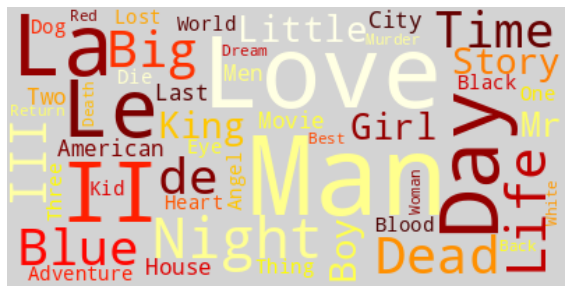Figure 2. Word Cloud.

Perhaps no particular surprises in the word cloud.

We’ll now create the schema for the movie postersas follows:

``````posters_schema = StructType([
StructField("id", IntegerType(), True),
StructField("poster", StringType(), True),
])``````

Create a new Dataframe, as follows:

``````posters_df = spark.read.csv(
"/FileStore/movie_poster.csv",
schema = posters_schema
)``````

Next, we’ll join the movies and posters Dataframes:

``````movies_df = movies_df.join(
posters_df,
["id"]
)``````

We can check the result as follows:

The output should be similar to this:

``````+---+--------------------+--------------------+--------------------+
| id|               title|              genres|              poster|
+---+--------------------+--------------------+--------------------+
|  1|    Toy Story (1995)|Animation|Childre...|https://m.media-a...|
|  3|Grumpier Old Men ...|      Comedy|Romance|https://m.media-a...|
|  4|Waiting to Exhale...|        Comedy|Drama|https://m.media-a...|
|  5|Father of the Bri...|              Comedy|https://m.media-a...|
+---+--------------------+--------------------+--------------------+
only showing top 5 rows``````

### Users

Let’s now turn to users. We’ll first provide the users schema, as follows:

``````users_schema = StructType([
StructField("id", IntegerType(), True),
StructField("gender", StringType(), True),
StructField("age", IntegerType(), True),
StructField("occupation_id", IntegerType(), True),
StructField("zip_code", StringType(), True)
])``````

Next, let’s read the users data into a Spark Dataframe:

``````users_df = spark.read.csv(
"/FileStore/users.dat",
schema = users_schema,
sep = separator
)``````

In the following code cell, we’ll take a look at the structure of the Dataframe:

The output should be similar to this:

``````+---+------+---+-------------+--------+
| id|gender|age|occupation_id|zip_code|
+---+------+---+-------------+--------+
|  1|     F|  1|           10|   48067|
|  2|     M| 56|           16|   70072|
|  3|     M| 25|           15|   55117|
|  4|     M| 45|            7|   02460|
|  5|     M| 25|           20|   55455|
+---+------+---+-------------+--------+
only showing top 5 rows``````

Let’s now perform a quick analysis of gender, as follows:

``````(users_df
.groupBy("gender")
.count()
.show()
)``````

The output shows that the users are approximately 70% male and 30% female:

``````+------+-----+
|gender|count|
+------+-----+
|     F| 1709|
|     M| 4331|
+------+-----+``````

Occupation is stored as a numeric value, but the mapping is described in the `README` file. We can create a list and schema as follows:

``````occupations = [( 0, "other"),
( 2, "artist"),
( 5, "customer service"),
( 6, "doctor/health care"),
( 7, "executive/managerial"),
( 8, "farmer"),
( 9, "homemaker"),
(10, "K-12 student"),
(11, "lawyer"),
(12, "programmer"),
(13, "retired"),
(14, "sales/marketing"),
(15, "scientist"),
(16, "self-employed"),
(17, "technician/engineer"),
(19, "unemployed"),
(20, "writer")]

occupations_schema = StructType([
StructField("occupation_id", IntegerType(), True),
StructField("occupation", StringType(), True)
])``````

Using this information, we can create a new Dataframe, as follows:

``````occupations_df = spark.createDataFrame(
data = occupations,
schema = occupations_schema
)``````

We can now join the users and occupations Dataframes so that we can get the correct occupation description and then drop any columns that we don’t need, as follows:

``````users_df = users_df.join(
occupations_df,
["occupation_id"]
)

users_df = users_df.drop(
"occupation_id"
)``````

Now, we’ll check the structure of the users Dataframe:

The output should be similar to this:

``````+----+------+---+--------+----------+
|  id|gender|age|zip_code|occupation|
+----+------+---+--------+----------+
|6039|     F| 45|   01060|     other|
|6031|     F| 18|   45123|     other|
|6023|     M| 25|   43213|     other|
|6019|     M| 25|   10024|     other|
|6010|     M| 35|   79606|     other|
+----+------+---+--------+----------+
only showing top 5 rows``````

We can also analyze the distribution of occupationsas follows:

``````(users_df
.groupBy("occupation")
.count()
.orderBy("count", ascending = False)
.show(21)
)``````

The output should be similar to this:

``````+--------------------+-----+
|          occupation|count|
+--------------------+-----+
|               other|  711|
|executive/managerial|  679|
| technician/engineer|  502|
|          programmer|  388|
|     sales/marketing|  302|
|              writer|  281|
|              artist|  267|
|       self-employed|  241|
|  doctor/health care|  236|
|        K-12 student|  195|
|           scientist|  144|
|             retired|  142|
|              lawyer|  129|
|    customer service|  112|
|           homemaker|   92|
|          unemployed|   72|
|              farmer|   17|
+--------------------+-----+``````

### Ratings

Next, let’s focus on ratings. We’ll first provide the ratings schema, as follows:

``````ratings_schema = StructType([
StructField("user_id", IntegerType(), True),
StructField("movie_id", IntegerType(), True),
StructField("rating", IntegerType(), True),
StructField("timestamp", IntegerType(), True)
])``````

Next, let’s read the ratings data into a Spark Dataframe:

``````ratings_df = spark.read.csv(
"/FileStore/ratings.dat",
schema = ratings_schema,
sep = separator
)``````

In the following code cell, we’ll take a look at the structure of the DataFrame:

The output should be similar to this:

``````+-------+--------+------+---------+
|user_id|movie_id|rating|timestamp|
+-------+--------+------+---------+
|      1|    1193|     5|978300760|
|      1|     661|     3|978302109|
|      1|     914|     3|978301968|
|      1|    3408|     4|978300275|
|      1|    2355|     5|978824291|
+-------+--------+------+---------+
only showing top 5 rows``````

We can get some additional information, as follows:

``````(ratings_df
.describe("user_id", "movie_id", "rating")
.show()
)``````

The output should be similar to this:

``````+-------+------------------+------------------+------------------+
|summary|           user_id|          movie_id|            rating|
+-------+------------------+------------------+------------------+
|  count|           1000209|           1000209|           1000209|
|   mean| 3024.512347919285|1865.5398981612843| 3.581564453029317|
| stddev|1728.4126948999715|1096.0406894572482|1.1171018453732606|
|    min|                 1|                 1|                 1|
|    max|              6040|              3952|                 5|
+-------+------------------+------------------+------------------+``````

We can see that the average movie rating appears quite generous.

We can generate a small rating histogram, as follows:

``````movie_ratings = ratings_df.select("rating").collect()
movie_ratings_list = [movie_ratings[i] for i in range(len(movie_ratings))]

plt.hist(
movie_ratings_list,
edgecolor = "white",
color = "#32B5C9",
rwidth = 0.9,
bins = [0.5, 1.5, 2.5, 3.5, 4.5, 5.5]
)

plt.ylabel("Frequency")
plt.xlabel("Rating")
plt.show()``````

The result should be similar to Figure 3.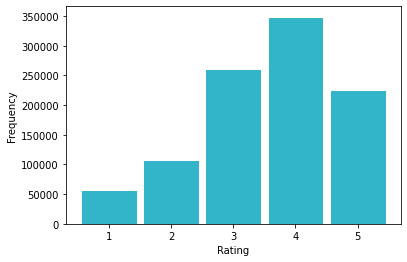Figure 3. Rating Histogram.

## Alternating Least Squares (ALS)

Let’s start by creating our train and test sets, as follows:

``(train, test) = ratings_df.randomSplit([0.7, 0.3], seed = 123)``

We’ll now use Spark’s ALS implementation to create our model:

``````from pyspark.ml.recommendation import ALS

als = ALS(
maxIter = 5,
regParam = 0.01,
userCol = "user_id",
itemCol = "movie_id",
ratingCol = "rating",
coldStartStrategy = "drop",
seed = 0
)

model = als.fit(train)``````

We can then show the predictions on the test data, as follows:

``````from pyspark.ml.evaluation import RegressionEvaluator

predictions = model.transform(test)

predictions.show(5)``````

The output should be similar to this:

``````+-------+--------+------+---------+----------+
|user_id|movie_id|rating|timestamp|prediction|
+-------+--------+------+---------+----------+
|    148|       1|     5|977335193| 4.3501983|
|    148|       2|     5|979578366| 3.8232095|
|    148|      11|     5|977334939|  4.321706|
|    148|      50|     2|979577217| 3.8569245|
|    148|      60|     3|979578136| 3.6407182|
+-------+--------+------+---------+----------+
only showing top 5 rows``````

We can determine the RMSE as follows:

``````re = RegressionEvaluator(
predictionCol = "prediction",
labelCol = "rating",
metricName = "rmse"
)

rmse = re.evaluate(predictions)
print(rmse)``````

The result should be similar to this:

We can view the user factors as follows:

``model.userFactors.show(5) # show(5, False) to show the whole column``

The output should be similar to this:

``````+---+--------------------+
| id|            features|
+---+--------------------+
| 10|[0.8803474, 0.799...|
| 20|[0.47382107, 0.65...|
| 30|[0.8101255, -0.38...|
| 40|[-0.008799124, 0....|
| 50|[-0.33016467, 0.4...|
+---+--------------------+
only showing top 5 rows``````

For example, the first line (`id = 10`) would be as follows:

``[0.8803474, 0.7996457, -1.3396101, 1.8472301, 0.7709434, 1.4858658, 0.5260316, 1.5704685, 0.14094345, 0.022391517]``

Similarly, we can view the item (movie) factors as follows:

``model.itemFactors.show(5) # show(5, False) to show the whole column``

The output should be similar to this:

``````+---+--------------------+
| id|            features|
+---+--------------------+
| 10|[0.08083672, 0.60...|
| 20|[0.5918954, 0.415...|
| 30|[-0.047557604, -0...|
| 40|[-1.0247108, 1.19...|
| 50|[0.1312179, -0.08...|
+---+--------------------+
only showing top 5 rows``````

For example, the first line (`id = 10`) would be as follows:

``[0.08083672, 0.60175675, -0.34102643, 0.4448118, 0.81003183, 0.50796205, 0.5284808, 0.009832226, 0.2445135, 0.1576684]``

Now that we have these two different vectors, we can store them in SingleStore DB. However, to do this, we need to convert them to a format that SingleStore DB understands. The SingleStore DB documentation provides guidance on acceptable vector formats.

First, let’s create two Dataframes, as follows:

``````user_factors_df = model.userFactors
item_factors_df = model.itemFactors``````

Next, let’s create and register a UDF with Spark that will perform the data conversion for us:

``````import array, binascii

def vector_to_hex(vector):
vector_bytes = bytes(array.array("f", vector))
vector_hex = binascii.hexlify(vector_bytes)
vector_string = str(vector_hex.decode())
return vector_string

vector_to_hex = udf(vector_to_hex, StringType())
spark.udf.register("vector_to_hex", vector_to_hex)``````

Let’s see how this UDF works with an example. Let’s take some data from earlier:

``vector = [0.8803474, 0.7996457, -1.3396101, 1.8472301, 0.7709434, 1.4858658, 0.5260316, 1.5704685, 0.14094345, 0.022391517]``

Using the following line:

``vector_bytes = bytes(array.array("f", vector))``

We will convert our above vector to the following representation:

``b'r^a?x95xb5L?Xxxabxbftrxec?x8c\E?xda0xbe?x02xaax06?x1dx05xc9?{Sx10>jnxb7<'``

The next line:

``vector_hex = binascii.hexlify(vector_bytes)``

Will then convert the previous output to the following representation:

``b'725e613f95b54c3f5878abbf0972ec3f8c5c453fda30be3f02aa063f1d05c93f7b53103e6a6eb73c'``

Finally, the following line:

``vector_string = str(vector_hex.decode())``

Will give us the data in the format that we can store in SingleStore DB:

``725e613f95b54c3f5878abbf0972ec3f8c5c453fda30be3f02aa063f1d05c93f7b53103e6a6eb73c``

We can now apply the UDF to the two Dataframes, as follows:

``````user_factors_df = user_factors_df.withColumn(
"factors",
vector_to_hex("features")
)

item_factors_df = item_factors_df.withColumn(
"factors",
vector_to_hex("features")
)``````

We can now join the users and movies Dataframes with their respective factors Dataframes and then drop any columns that we don’t need. First, users:

``````users = users_df.join(
user_factors_df,
["id"]
)

users = users.drop("features")``````

Next, movies:

``````movies = movies_df.join(
item_factors_df,
["id"]
)

movies = movies.drop("features")``````

## Write Data To SingleStore DB

We are now ready to write the users and movies Dataframes to SingleStore DB. In the following code cell, we can add the following:

In the Setup notebook, we need to ensure that the server address and password have been added for our SingleStore Cloud cluster.

In the following code cell, we’ll set some parameters for the SingleStore Spark Connector, as follows:

``````spark.conf.set("spark.datasource.singlestore.ddlEndpoint", cluster)
spark.conf.set("spark.datasource.singlestore.disablePushdown", "false")``````

Finally, we are ready to write the Dataframes to SingleStore DB using the Spark Connector. First, users:

``````(users.write
.format("singlestore")
.mode("ignore")
.save("recommender_db.users")
)``````

Next, movies:

``````(movies.write
.format("singlestore")
.mode("ignore")
.save("recommender_db.movies")
)``````

We can check that the two database tables were successfully populated from SingleStore Cloud.

## Example Queries

Now that we have built our system, we can run some queries. Let’s include the three example queries from the article referenced earlier.

First, let’s make movie recommendations for the user with `id = 1`as follows:

``````SELECT movies.title,
movies.genres,
DOT_PRODUCT(UNHEX(users.factors), UNHEX(movies.factors)) AS score
FROM users JOIN movies
WHERE users.id = 1
ORDER BY score DESC
LIMIT 10;``````

This produces the following output shown in Figure 4: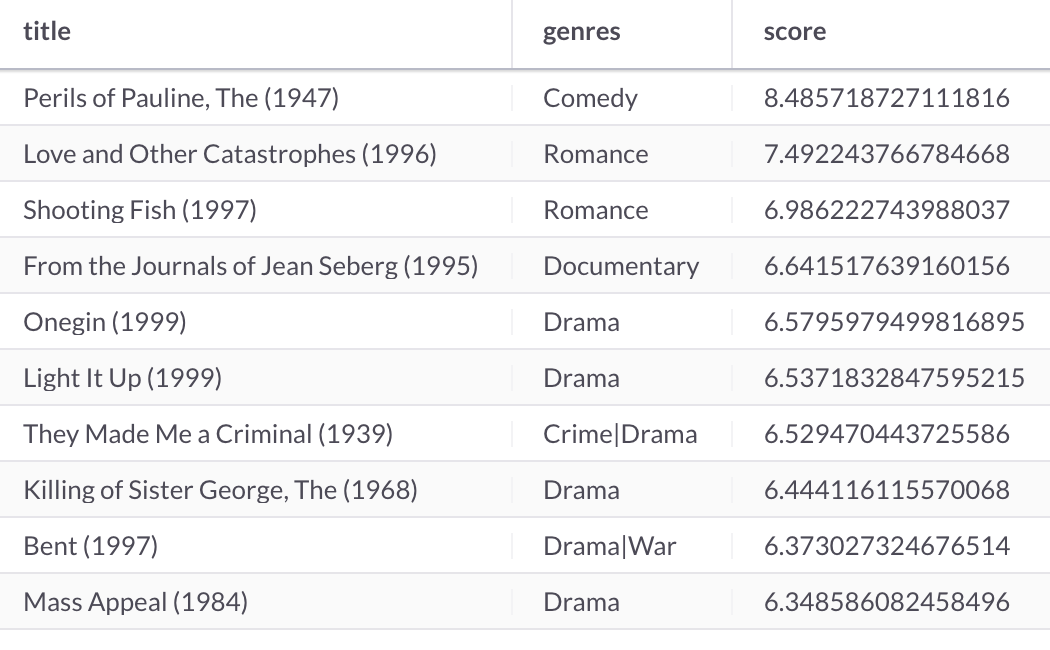Figure 4. Movie Recommendations.

We can also make user recommendations for the movie with `id = 1`as follows:

``````SELECT users.gender,
users.age,
users.occupation,
users.zip_code,
DOT_PRODUCT(UNHEX(movies.factors), UNHEX(users.factors)) AS score
WHERE movies.id = 1
ORDER BY score DESC
LIMIT 10;``````

This produces the following output shown in Figure 5: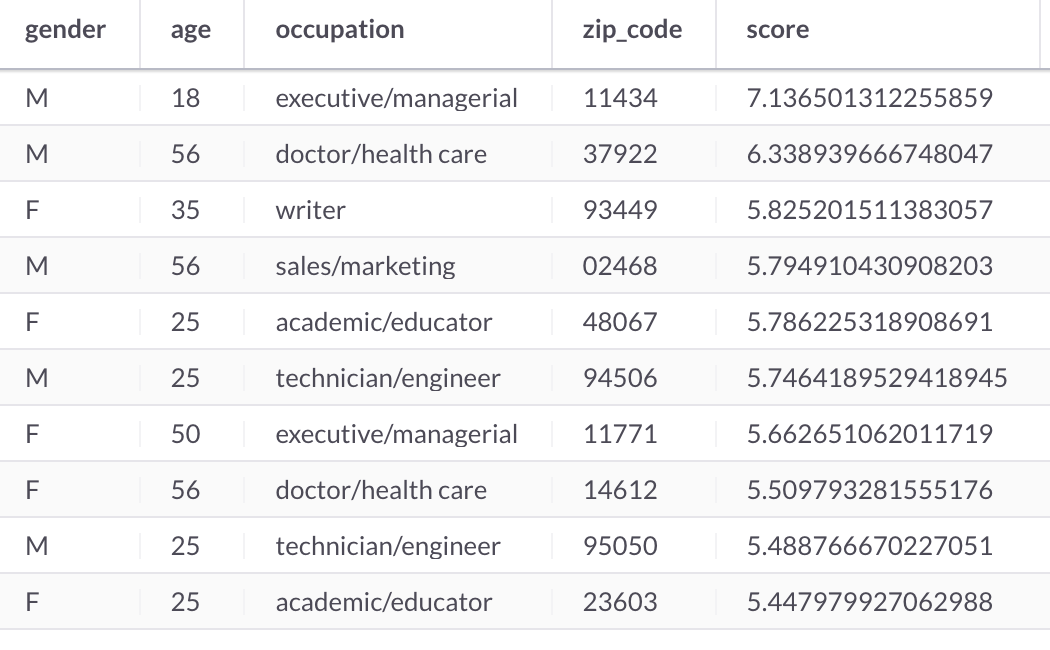Figure 5. User Recommendations.

Next, let’s make Sci-Fi movie recommendations for the 1980s for the same user, as follows:

``````SELECT movies.title,
movies.genres,
DOT_PRODUCT(UNHEX(users.factors), UNHEX(movies.factors)) AS score
FROM users JOIN movies
WHERE users.id = 1 AND
SUBSTRING(movies.title, -3, 1) = 8 AND
movies.genres LIKE '%sci-fi%'
ORDER BY score DESC
LIMIT 10;``````

This produces the following output shown in Figure 6: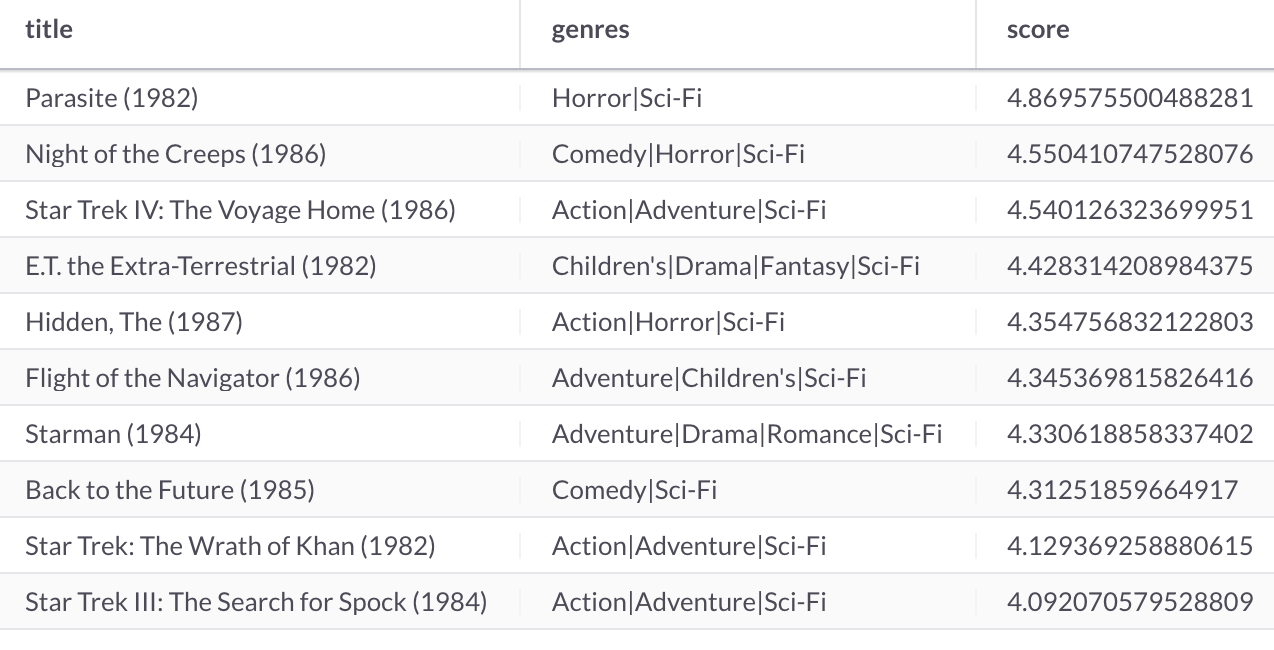Figure 6. Movie Recommendations.

Finally, let’s make movie recommendations for a new Male user that is within the 18-24 age range, as follows:

5 GROUP BY 1, 2 ORDER BY avg_score DESC LIMIT 10;” data-lang=”text/x-sql”>

``````SELECT movies.title,
movies.genres,
AVG(DOT_PRODUCT(UNHEX(users.factors), UNHEX(movies.factors))) AS avg_score,
count(1) AS c
FROM users JOIN movies
WHERE users.gender="M" AND
users.age = 18 AND
DOT_PRODUCT(UNHEX(users.factors), UNHEX(movies.factors)) > 5
GROUP BY 1, 2
ORDER BY avg_score DESC
LIMIT 10;``````

This produces the following output shown in Figure 7: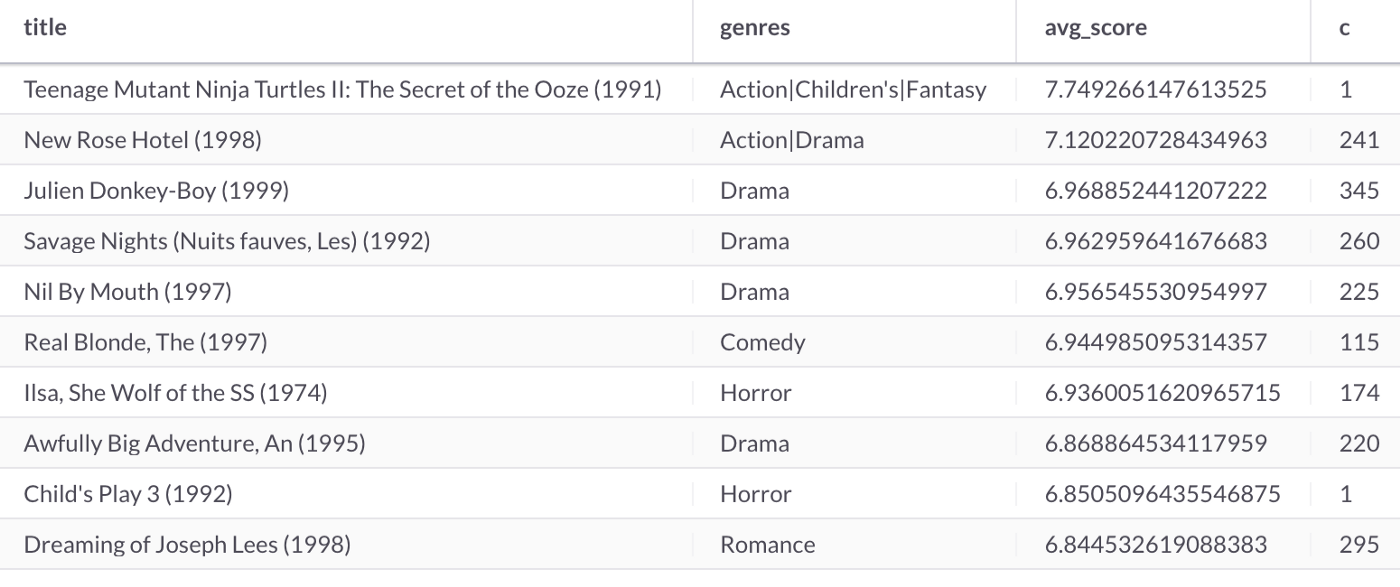Figure 7. Movie Recommendations.

## Bonus: Streamlit Visualization

We can build a small application for the first query above and render images for movie posters. We can do this quite easily with Streamlit. A previous article showed the ease with which we could connect Streamlit to SingleStore DB.

### Install the Required Software

We need to install the following packages:

These can be found in the `requirements.txt` file on GitHub. Run the file as follows:

``pip install -r requirements.txt``

### Example Application

Here is the complete code listing for `streamlit_app.py`:

``````# streamlit_app.py
import streamlit as st
import pandas as pd
import pymysql

# Initialize connection.

def init_connection():
return pymysql.connect(**st.secrets["singlestore"])

conn = init_connection()

user_id = st.sidebar.number_input("Enter a User Id", min_value = 1, max_value = 6040)

# Perform query.

SELECT movies.title,
movies.poster,
DOT_PRODUCT(UNHEX(users.factors), UNHEX(movies.factors)) AS score
FROM users JOIN movies
WHERE users.id = %s
ORDER BY score DESC
LIMIT 10;
""", conn, params = ([str(user_id)]))

for i in range(10):
cols = st.columns(1)
cols.image(data["poster"][i], width = 200)``````

### Create a Secrets File

Our local Streamlit application will read secrets from a file `.streamlit/secrets.toml` in our application’s root directory. We need to create this file as follows:

``````# .streamlit/secrets.toml

[singlestore]
host = "<TO DO>"
port = 3306
database = "recommender_db"

The `<TO DO>` for host and password should be replaced with the values ​​obtained from SingleStore Cloud when creating a cluster.

### Run the Code

We can run the Streamlit application as follows:

``streamlit run streamlit_app.py``

The output in a web browser should look like Figure 8.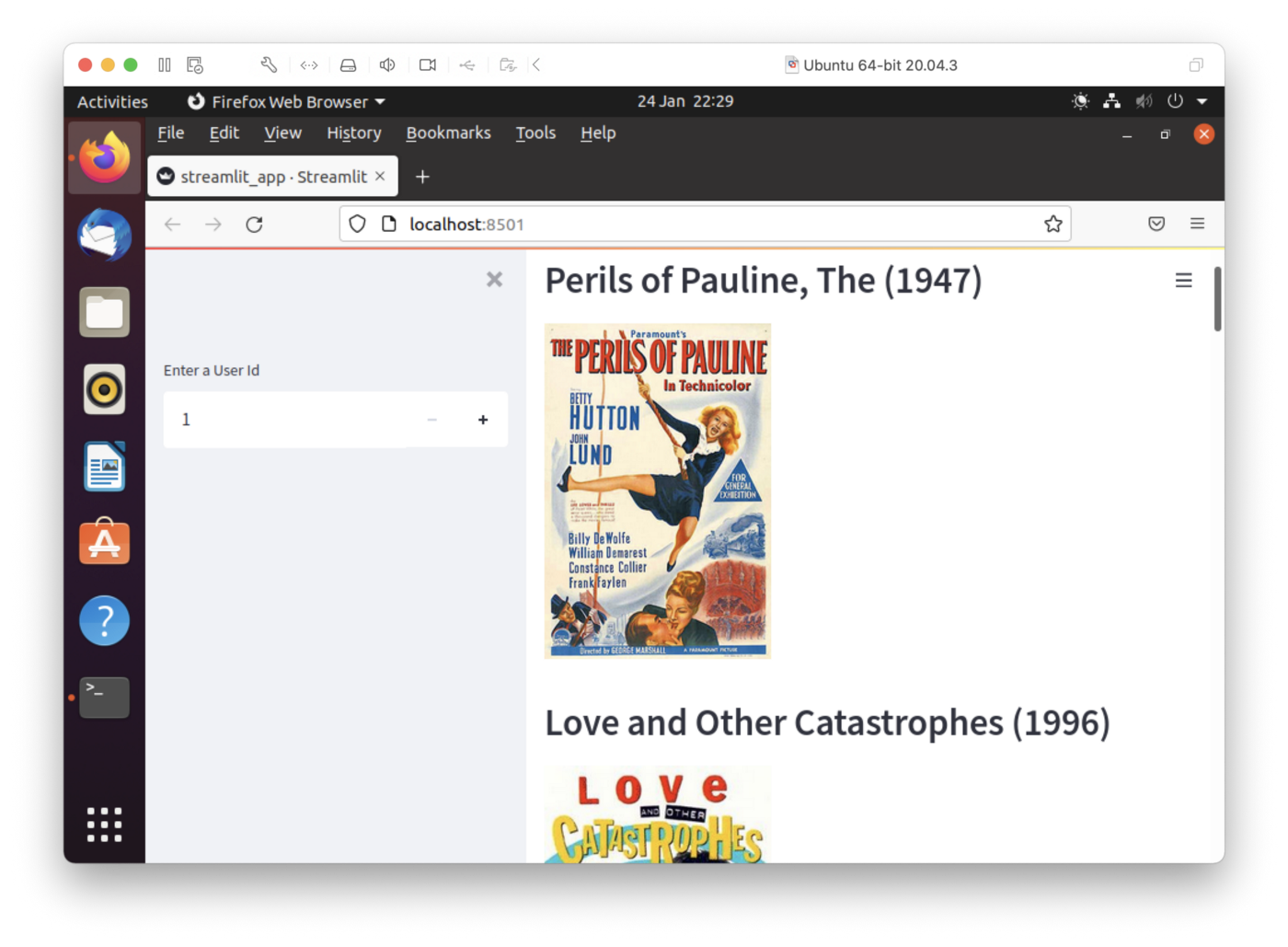Figure 8. Streamlit.

We can enter a new user id in the input box on the left-hand side of the web page. The top 10 movie recommendations are shown on the right-hand side. Feel free to experiment with the code to suit your needs.

## Summary

This article has built a movie recommender system using SingleStore DB, Spark, and Alternating Least Squares. We have seen how we can store vectors in SingleStore DB and using the built-in functions, `DOT_PRODUCT` and `UNHEX`, we can perform operations on those vectors directly in the database system. We can make movie recommendations for existing users and predictions for new users using these built-in functions.

.